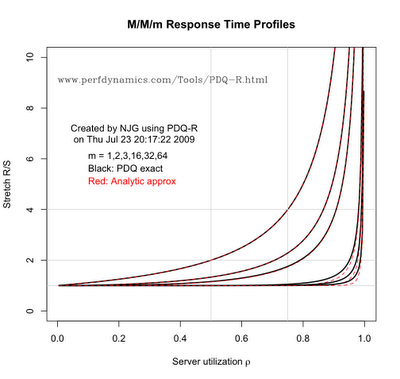## Wednesday, July 29, 2009

### Remembering Mr. Erlang as a Unit

Not to be confused with Frank Zappa's daughter or a Coneheads spousal unit, is the Erlang unit. The number of Erlangs (E) is defined as:

 E  =  λ S, (1)

and is a well-known in the teletraffic industry, where it was first used as a measure of call capacity. For this reason, it's also called the traffic intensity.

In the context of the M/M/m queue, λ is the arrival rate (of telephone calls, for example), S is the time to service the call, and m is the number of servers or outgoing phone lines. The point about the Erlang unit is that you can't have more Erlangs (E) than you have server capacity (m) or the waiting line will become infinite, i.e., E < m. To keep things consistent, we have to ensure that none of the m servers exceeds 100% busy, i.e., ρ < 1. This requirement can be met by dividing E by m, so that the per-server utilization is:

 ρ < E/m,   or equivalently,   ρ < λ S/m. (2)

Compare this with the single server case (m = 1) where ρ < λ S; something I call Little's microscopic law (because it involves only the service time, S, not the response time, R). This is where the confusion comes in. If you think of Little's law, you have to remember to divide it by m for an M/M/m queue. No matter how many times I fool around with this stuff, Erlang always seems to find a way to get me.

My most recent scrape with this confusion arose when I tried to produce the following plot using the new multi-server queue capability in PDQ version 5.0, as defined in Chapter 6 of my Perl::PDQ book. This is the kind of queueing model that might be applied to assessing capacity or performance of multicore processors, threaded apps, etc., as long as there is no significant sharing between the servers.Fig. 1 Correct curves

In particular, I was using PDQ-R variant PDQ. One of the nice things about R is its integrated graphics. The uppermost curve is for a single server (m = 1), and the lowest curve corresponds to m = 64-way servers. The red dashed lines are produced by an approximate analytic formula as a cross-check. However, when I ran the PDQ-R code it generated the following plot.Fig. 2 Incorrect curves

As you can see, except for the single-server case (m = 1), the solutions produced by PDQ-R (black curves) do not even come close to matching the analytic solutions. Moreover, as the number of servers (m) is increased, the PDQ response times remain rather flat and never go to infinity near 100% server utilization. In a word, it's wrong! This is disturbing, because I just put together a test suite to check that the multi-server capability worked correctly. When I checked those tests again, the PDQ numerics were correct. The only difference was that the test suite didn't generate plots.

To produce the curves in the utilization range: 0 < ρ < 1, I needed to increment the arrival rate inside a loop, and I chose a delta of 0.01 to do that. Now, in PDQ things are specified a bit differently from the way you go about it with the usual M/M/m queueing theory math. The arrival rate, arate, is a parameter for CreateOpen(), whereas the service time, stime, at the multi-server is a SetDemand() parameter. So, if the loop over the number of servers looks something like this:
for (m in servers) {   ...   arate <- arate + 0.01   CreateOpen(work, arate)   CreateMultiNode(m, node, CEN, FCFS)   SetDemand(node, work, stime)   ...}

you end up with the wrong curves shown above. What's gone wrong?

Notice that the x-axis of the plots is the per-server utilization (ρ). So, a vertical line that drops through the response time curves at a given ρ value (e.g., the vertical line at ρ = 0.75 in the first plot), corresponds to increasing the traffic volume (λ) on each successive curve. The thing to remember is: as you add more server capacity (m), you need to add an equivalent amount of work (in Erlangs) to keep each server at the same utilization. The simple fix in PDQ-R is:
for (m in servers) {   ...   arate <- arate + 0.01   CreateOpen(work, m * arate)   CreateMultiNode(m, node, CEN, FCFS)   SetDemand(node, work, stime)   ...}

In other words, you have to multiply the base arrival rate by the number of servers (m) to match the increased server capacity in Erlang units. Relative to the definition in (1), this looks counter-intuitive. That's partly because of the way things need to be set up in PDQ. Moreover, nothing is really wrong with the second plot. Rather, it correctly shows what happens if you just add more server capacity for the same volume of traffic. With all that additional capacity, of course the response time remains close to the individual service time, and therefore looks flat.

Now, you see how Mr. Erlang can get you, no matter how much of a queueing-Conehead you think you are.